# VLOOKUP

The VLOOKUP function is one of the most popular functions in Excel. This page contains many easy to follow VLOOKUP examples.

### Exact Match

Most of the time you are looking for an exact match when you use the VLOOKUP function in Excel. Let's take a look at the arguments of the VLOOKUP function.

1. The VLOOKUP function below looks up the value 53 (first argument) in the leftmost column of the red table (second argument).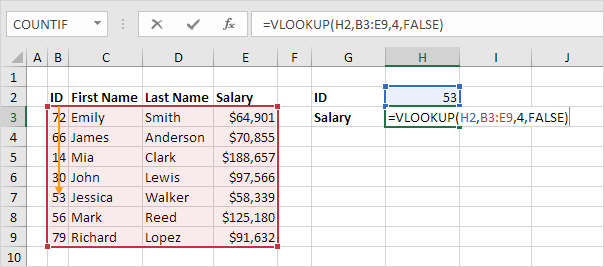2. The value 4 (third argument) tells the VLOOKUP function to return the value in the same row from the fourth column of the red table.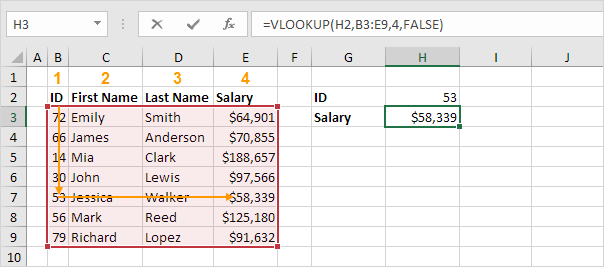Note: the Boolean FALSE (fourth argument) tells the VLOOKUP function to return an exact match. If the VLOOKUP function cannot find the value 53 in the first column, it will return a #N/A error.

3. Here's another example. Instead of returning the salary, the VLOOKUP function below returns the last name (third argument is set to 3) of ID 79.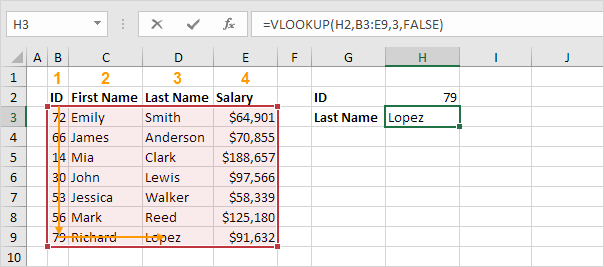### Approximate Match

Let's take a look at an example of the VLOOKUP function in approximate match mode (fourth argument set to TRUE).

1. The VLOOKUP function below looks up the value 85 (first argument) in the leftmost column of the red table (second argument). There's just one problem. There's no value 85 in the first column.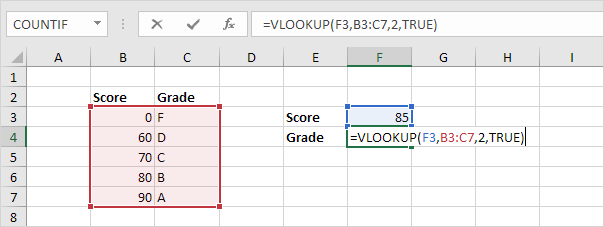2. Fortunately, the Boolean TRUE (fourth argument) tells the VLOOKUP function to return an approximate match. If the VLOOKUP function cannot find the value 85 in the first column, it will return the largest value smaller than 85. In this example, this will be the value 80.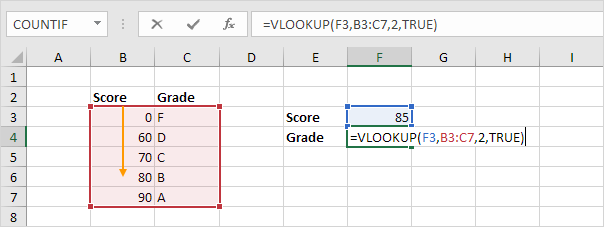3. The value 2 (third argument) tells the VLOOKUP function to return the value in the same row from the second column of the red table.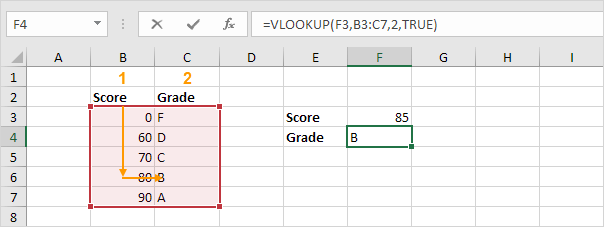Note: always sort the leftmost column of the red table in ascending order if you use the VLOOKUP function in approximate match mode (fourth argument set to TRUE).

### Vlookup Looks Right

The VLOOKUP function always looks up a value in the leftmost column of a table and returns the corresponding value from a column to the right.

1. For example, the VLOOKUP function below looks up the first name and returns the last name.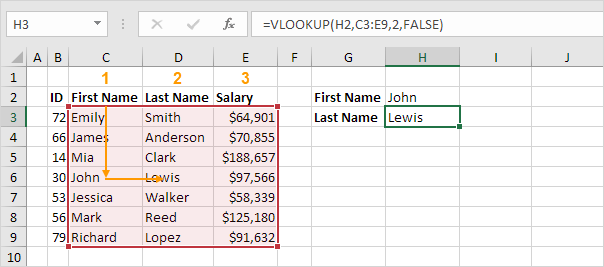2. If you change the column index number (third argument) to 3, the VLOOKUP function looks up the first name and returns the salary.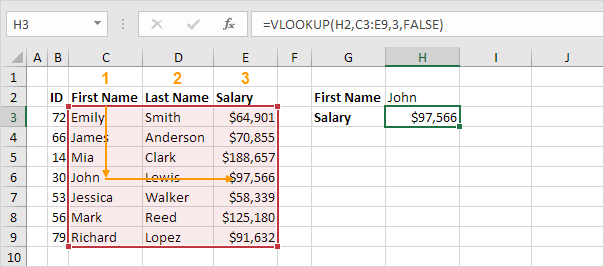Note: in this example, the VLOOKUP function cannot lookup the first name and return the ID. The VLOOKUP function only looks to the right. No worries, you can use INDEX and MATCH in Excel to perform a left lookup.

### First Match

If the leftmost column of the table contains duplicates, the VLOOKUP function matches the first instance. For example, take a look at the VLOOKUP function below.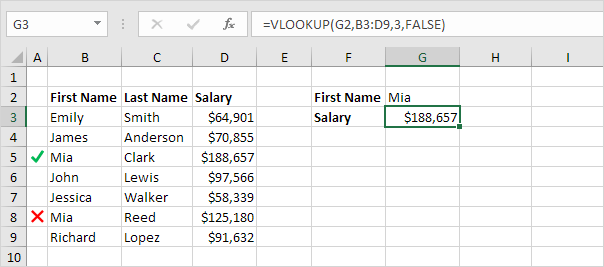Explanation: the VLOOKUP function returns the salary of Mia Clark, not Mia Reed.

### Partial Match

The VLOOKUP function supports wildcards for partial matches. For example, take a look at the VLOOKUP function below.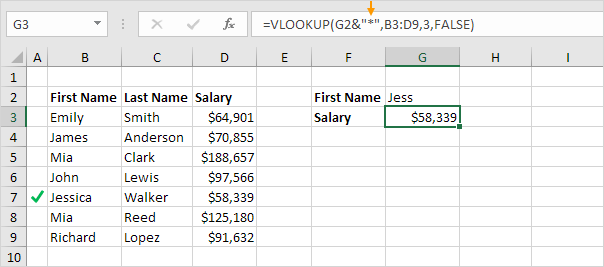Explanation: an asterisk (*) matches zero or more characters. The VLOOKUP function matches the first instance that begins with "Jess". Use "*"&G2 for an "ends with" match. Use "*"&G2&"*" for a "contains" match.

### Vlookup is Case-insensitive

The VLOOKUP function in Excel performs a case-insensitive lookup. For example, the VLOOKUP function below looks up MIA (cell G2) in the leftmost column of the table.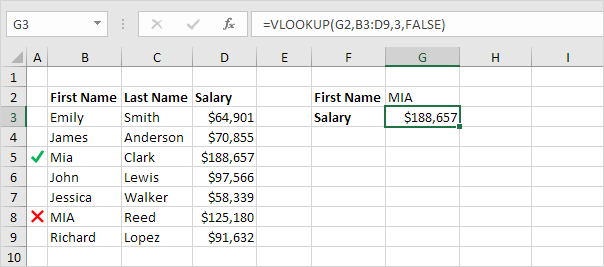Explanation: the VLOOKUP function is case-insensitive so it looks up MIA or Mia or mia or miA, etc. As a result, the VLOOKUP function returns the salary of Mia Clark (first instance). Use INDEX, MATCH and EXACT in Excel to perform a case-sensitive lookup.

### Multiple Criteria

Do you want to look up a value based on multiple criteria? Use INDEX and MATCH in Excel to perform a two-column lookup.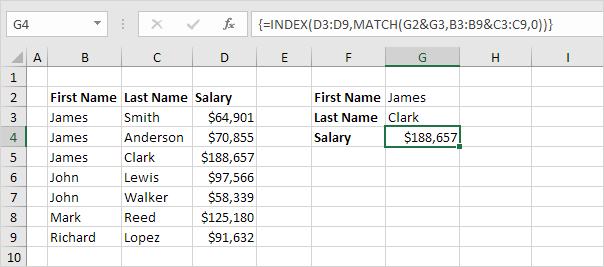Note: the array formula above looks up the salary of James Clark, not James Smith, not James Anderson.

### #N/A error

If the VLOOKUP function cannot find a match, it returns a #N/A error.

1. For example, the VLOOKUP function below cannot find the value 28 in the leftmost column.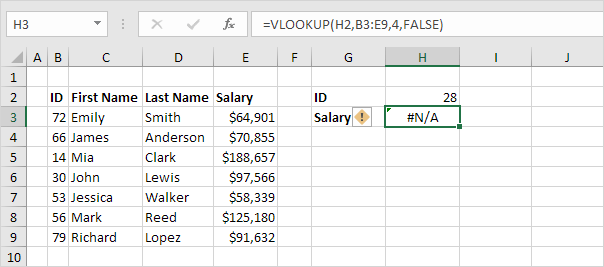2. If you like, you can use the IFNA function to replace the #N/A error with a friendly message.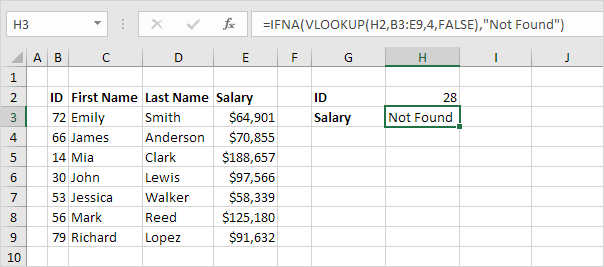Note: the IFNA function was introduced in Excel 2013. If you're using Excel 2010 or Excel 2007, combine IF and ISNA or use IFERROR.

### Multiple Lookup Tables

When using the VLOOKUP function in Excel, you can have multiple lookup tables. You can use the IF function to check whether a condition is met, and return one lookup table if TRUE and another lookup table if FALSE.

1. Create two named ranges: Table1 and Table2.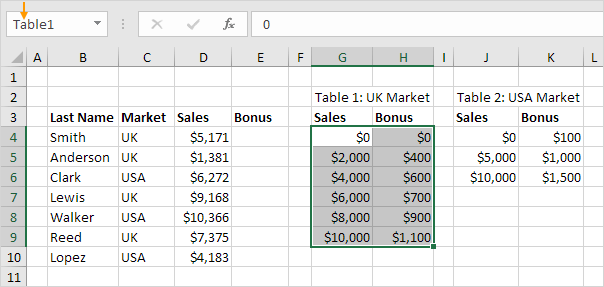2. Select cell E4 and enter the VLOOKUP function shown below.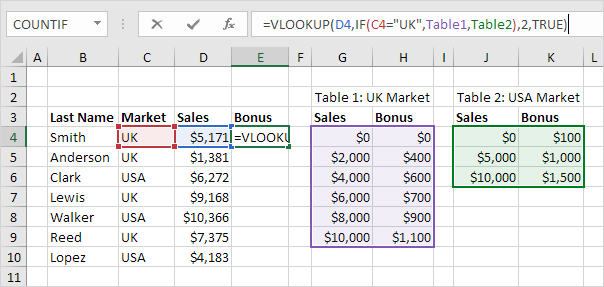Explanation: the bonus depends on the market (UK or USA) and the sales amount. The second argument of the VLOOKUP function does the trick. If UK, the VLOOKUP function uses Table1, if USA, the VLOOKUP function uses Table2. Set the fourth argument of the VLOOKUP function to TRUE to return an approximate match.

3. Press Enter.

4. Select cell E4, click on the lower right corner of cell E4 and drag it down to cell E10.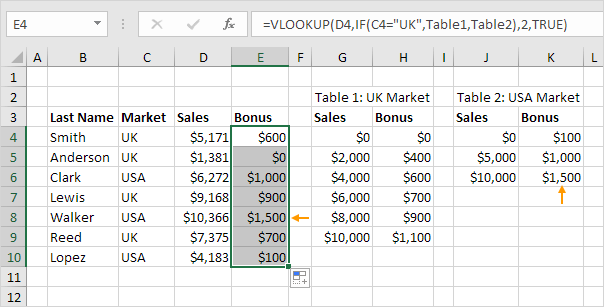Note: for example, Walker receives a bonus of \$1,500. Because we're using named ranges, we can easily copy this VLOOKUP function to the other cells without worrying about cell references.

### Index and Match

Instead of using VLOOKUP, use INDEX and MATCH. To perform advanced lookups, you'll need INDEX and MATCH. Maybe this is one step too far for you at this stage, but it shows you one of the many other powerful formulas Excel has to offer.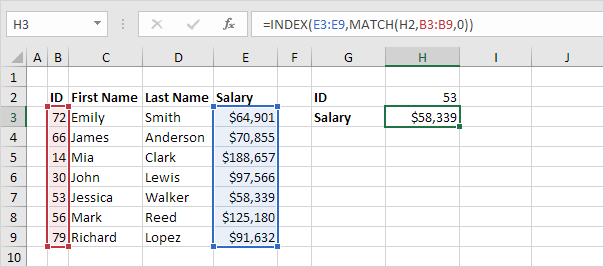### Table Magic

Tables can make VLOOKUP formulas a lot easier. In the example below, a single VLOOKUP function automatically looks up all country codes.Note: visit our page about merging tables to learn how to create this VLOOKUP function and bring your Excel game to a new level.

### Xlookup

If you have Excel 365 or Excel 2021, use XLOOKUP instead of VLOOKUP. The XLOOKUP function is easier to use and has some additional advantages.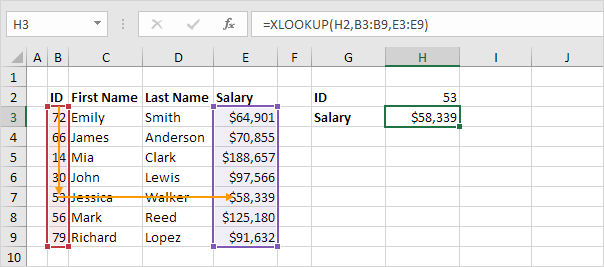Go to Next Chapter: Financial Functions